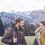# Proof Contest Day 1

Prove that there no solutions for

$\large{5^a+8^b=c^2 }$

For $a,b,c \in \mathbb{Z^{+}}$ and $b$ is not an even positive integer.Note by Department 8
5 years, 4 months ago

This discussion board is a place to discuss our Daily Challenges and the math and science related to those challenges. Explanations are more than just a solution — they should explain the steps and thinking strategies that you used to obtain the solution. Comments should further the discussion of math and science.

When posting on Brilliant:

• Use the emojis to react to an explanation, whether you're congratulating a job well done , or just really confused .
• Ask specific questions about the challenge or the steps in somebody's explanation. Well-posed questions can add a lot to the discussion, but posting "I don't understand!" doesn't help anyone.
• Try to contribute something new to the discussion, whether it is an extension, generalization or other idea related to the challenge.

MarkdownAppears as
*italics* or _italics_ italics
**bold** or __bold__ bold
- bulleted- list
• bulleted
• list
1. numbered2. list
1. numbered
2. list
Note: you must add a full line of space before and after lists for them to show up correctly
paragraph 1paragraph 2

paragraph 1

paragraph 2

[example link](https://brilliant.org)example link
> This is a quote
This is a quote
    # I indented these lines
# 4 spaces, and now they show
# up as a code block.

print "hello world"
# I indented these lines
# 4 spaces, and now they show
# up as a code block.

print "hello world"
MathAppears as
Remember to wrap math in $$ ... $$ or $ ... $ to ensure proper formatting.
2 \times 3 $2 \times 3$
2^{34} $2^{34}$
a_{i-1} $a_{i-1}$
\frac{2}{3} $\frac{2}{3}$
\sqrt{2} $\sqrt{2}$
\sum_{i=1}^3 $\sum_{i=1}^3$
\sin \theta $\sin \theta$
\boxed{123} $\boxed{123}$

Sort by:

yes it must have i;e solve by indicies a+b=2

- 5 years, 3 months ago

The bases are not same. Additionally, there's no multiplication involved so you cannot add the indices.

- 5 years, 3 months ago

$c^2 \equiv 0, 1, 4, 9, 6, 5 \pmod{10}$

$5^a \equiv 5 \pmod{10}$

$8^b \equiv 8, 2 \pmod{10} (b \text{ is odd})$

$5^a + 8^b \equiv 3,7 \pmod{10}$

Clearly, the first statement and the last statement share no residues. Thus proven.

- 5 years, 4 months ago

What's mod? And how are 6 and 5 also included?

- 5 years, 3 months ago

$a \equiv b \pmod c$

Implies a leaves same remainder as b when divided by c.

- 5 years, 3 months ago

Haha, the same way I solved the question!

- 5 years, 4 months ago

Nice problem. By the way , from where have you collected these problems?

- 5 years, 4 months ago

Own thinking. Try other problems too from this set.

- 5 years, 4 months ago

Trying others.

- 5 years, 4 months ago